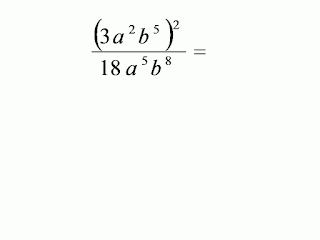## 26 May 2012

### Indices and Expanding Algebraic Expressions

1.         Simplify m3 ×m5

2.         k8 × k4 =
3.         Find the value of ( 32 )2
4.         ( – 6n3 )4 ÷ (2n2)3 =

5.6.         Simplify ( 4ac2 )3 × 2a– 1

7.         Simplify  p11 ÷ ( p5 × p ).

8.         Evaluate ( 3– 2 )2 × 92

9.        Given that  32m – 1 = 35, find the value of m.

10.       Given that ( 53 )x = 59, find the value of x.

11.       Expand the following
(a)       3(m + 6)
(b)       – 4 (2 – 3x + 5r)

12.       Expand the following
(a)       (y – 2)(y – 6)
(b)       (p + 2)(p – 5)

13.       Expand the following
(a)       (1 – 4n)2
(b)       5(2m + 3)2